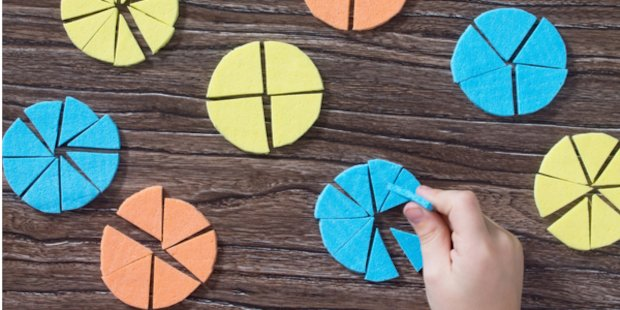# Mastery in Mathematics – Fractions, Decimals and Percentages Recommended 5 Stars

This item has 5 stars of a maximum 5

Rated 5/5 from 1 rating (Write a review)

Mastery in mathematics is as much about making the links between aspects of mathematics as it is about deepening understanding of a particular concept. It is clear that to do the former, the latter has to be in place, and a security of conceptual understanding must be present. If not, then methods will continue to be understood in isolation of one another.The use and manipulation of fractions, decimals and percentages form one of the most complex and abstract areas of number that primary age children experience. If we understand the main reasons why children struggle in this area (see list below), then we can plan to ensure that we are fully supporting our pupils’ needs.

Reasons why children struggle in this area
• It conflicts with what children learn initially about the property of number. Until they come across a fraction, their understanding of counting up and down and knowledge of place value is fixed on ‘the bigger the number the bigger the value’. For example, 4 is bigger than 3. However, when a numerator and denominator are introduced as a fraction, we see that we are now dealing with ‘parts of a whole’ – 13 is a bigger amount than 14 because of the number of parts any object has been split into.
• Knowing times tables and their relationship is fundamental to understanding fractional equivalence. For example, the relationship between doubling and halving numbers with the 2 times table helps enormously when identifying that 12 = 24. Therefore, not having a secure knowledge of time tables can greatly affect a child’s ability to understand fractions.
• Developing the relationship between certain times tables (5 and 10, 2, 4, 6 and 8, 3, 6 and 9) allows for links to be made in respect of equivalence. However, it can be hard for children to see these abstract links and adding ‘parts of a whole’ complicates it more.
• Moving too quickly to abstract thinking when teaching fractions can confuse children. Spending more time on concrete and pictorial teaching methods ensures that the basic stepping stones are in place before you move onto more abstract ideas. It is sometimes assumed that once a child reaches around 9 years old, if they are competent mathematicians, the use of manipulatives is unnecessary. However, research shows that abstract understanding is not the norm until the pre-adolescent years, meaning gaps can occur in real understanding simply because a concept has not been thoroughly understood.
• The interrelationship between fractions, decimals and percentages is made harder by teaching them in isolation. We often approach the delivery of these three areas separately in order to ensure the children can utilise methods that are taught for each. However, teaching them together allows for links to be made and efficiency to occur. One child may find it easier to convert into fractions, another decimals in order to work out calculations.

### Progression in Fractions, Decimals and Percentages

Fractions:
Children need to:
• Understand and identify halves (12) and then quarters (14) as two/four parts to show equal sharing/grouping.
• Once security is gained move on to other unit fractions (where the numerator is 1) with small denominators.
• Moving to 24, 34, 23 etc. enables further development. 24 and 34 are often the first non-unit fractions.
• Order/compare on numberlines (often starting from 0-1 then moving to greater numbers). This will develop understanding.
• Count in different fractional steps to further develop this understanding of fractions as numbers, ensuring they can see that fractions can be greater than 1.
• Develop understanding of linearity, eg 12, 1, 112, 2 etc. This enables children to position fractions on numberlines, as well as finding the term and rule of a sequence.
• Develop an understanding of simple equivalence, eg 12= 24 before moving onto looking at other fractions with larger denominators.
• Understanding of equivalence using denominators of greater numerical size, eg 23, 46 etc. before using their knowledge of factors and multiples to deduce equivalence and simplify fractions is important.
• Finding the lowest common denominator to help with equivalence and comparison, eg 69, 1827 = 23.
• Develop an understanding that fractions are operators, eg find a fraction of a quantity (or measure).
• Simple fractions of amounts, eg 12 of 4 = 2 (unit fractions) underpin later understanding of finding larger fractions and non-unit fractions of amounts.
• Relating finding fractions of amounts to division is important.
• Develop an understanding of fractions in calculations.
• It is important to add fractions to 1 before larger numbers to aid understanding.
• Fluency in adding and subtracting fractions with same denominator must be in place before different denominators are introduced.
• Following this, using mixed and improper fractions and converting between them should occur. Developing that into addition and subtraction calculations, along with finding the simplest form of the answer.
• Multiplication and division of fractions should be proper fractions and mixed numbers by whole numbers, so as to see what effect multiplication and division have when using values less than 1.
Member-only content

## Scholastic Resource Bank: Primary - join today!

• Over 6,000 primary activities, lesson ideas and resources
• Perfect for anyone working with children from 5 to 11 years old
• Unlimited access from just £1.25 per month